# Create Events

Combine channels and subcases to create events.

Tip: You can rename a created event by double-clicking on its names in the bottom half of the Load Map dialog.

## Create Time Series Events

Static subcases are supported for all fatigue calculations with a Time Series loading type.
1. Click the Load Map tool.
2. Using the bottom half of the dialog, specify how to create events then click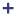.
The fatigue subcases that are read from the result file can be paired with load histories either manually or automatically.

All pairs will be superimposed.

Note: The number of time points should be the same if the pairs under the same event are superimposed.
Option Description
Manual Event Creation
When Manual is selected and you click, an event is created without any load history and subcase pair assigned. You can drag and drop an equal number of load histories and subcases under an event.
Note: The subcases should be dropped under an event first and then the load history channels.
Auto Event Creation
When Auto and Single Event are selected, before you click,
1. Select a subcase and a load history channel, or
2. Select multiple load histories and subcase pairs that have an equal number of time points.

Only one event is created when you click.

When Auto and Multiple Events are selected, before you click,
1. Select multiple load histories and subcase pairs.

The load histories must have an equal number of time points.

2. Total number of events created = Number of channels selected / Number of subcases selected.
3. Optional: Import an Event Mapper .csv.
1. Verify the Analysis type is set to Time Data.
2. In the Event definition area, click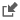and select the event definition .csv file.
Both FE Subcases and load history files should be present in the loadmap. On import of the Event Mapper .csv, FE subcases are automatically paired with load history channels present in the Loadmap. The CSV is organized by defining single or multiple Events based on the IDs of FE loadcases and load history channels. Load histories of the RPC/RSP format and multi-channel CSV are supported.
Restriction: The load history files must be available in the LoadMap context before importing an Event Mapper file.
4. Adjust the load magnitude, scale, offset, repeats, max gate range, and configuration of the events/subcases.
Tip:
• Apply the value of a parameter to all the events/subcases by right-clicking on a value and selecting Apply value to all events.
• From the Subcase window, select any subcase increment.
5. Activate the checkboxes of the events to be considered in the evaluation.
The following equation depicts how LDM, Scale, and Offset values work together to scale the FEA stress tensor at time t.(1) ${\sigma }_{ij}\left(t\right)=\frac{{\sigma }_{ij.FEA}}{LDM}\left(P\left(t\right)Scale+Offset\right)$
Where:
${\sigma }_{ij}\left(t\right)$
Results stress tensor at time t
${\sigma }_{ij.FEA}$
Stress tensor from static analysis
$P\left(t\right)$
The y point value of load-time history at time t

## Event Configuration: Superposition

The following equation depicts how LDM, Scale, and Offset values work together to scale the FEA stress tensor at time t, for the entire fatigue event:(2) $\left({\sigma }_{ij}\left(t\right)\right)event=\sum _{l=1}^{n}\left[\frac{{\left({\sigma }_{i{j}^{FEA}}\right)}_{l}}{LD{M}_{l}}\left(P{\left(t\right)}_{l}{\left(Scale\right)}_{l}+{\left(Offset\right)}_{l}\right)\right]$
Where:
$\left({\sigma }_{ij}\left(t\right)\right)event$
Superposed stress tensor for the entire fatigue event, as a function of time t.
$l$
The subcase and load history pair defined in the event, the number of pairs in an event varies from one to n.
${\left({\sigma }_{i{j}^{FEA}}\right)}_{l}$
Stress tensor from static analysis
$LD{M}^{}$
Magnitude used to scale the finite element stresses
$P{\left(t\right)}_{l}$
Point in load history at time t
${\left(Scale\right)}_{l}$
Scale factor applied to the load or time history. It is ignored in fatigue analysis based on a transient analysis subcase.
${\left(Offset\right)}_{l}$
Offset applied to the load or time history. It is ignored in fatigue analyses based on a transient analysis subcase or if the event configuration is sequential.

## Event Configuration: Sequential

If Sequential flag is selected in an event, all subcases referenced in that event are applied sequentially, instead of simultaneously. The resulting stress history is generated by the subcases present in the event, each subcase is considered sequential at different time points. Therefore, subcase1 at t1, subcase2 at t2, and so on.

The stress tensors at each time t from each subcase is calculated as:(3) ${\sigma }_{i{j}^{\left(t\right)}}=\frac{{\sigma }_{i{j}^{FEA}}}{LD{M}^{}}$
Where:
${\sigma }_{{i}_{j}}\left(t\right)$
Stress tensor for the fatigue load, as a function of time t
${\sigma }_{i{j}^{FEA}}$
Stress tensor from static analysis
$LD{M}^{}$
Magnitude used to scale the finite element stresses

## Event Configuration: Range Envelope

With Range envelope selection for an event, the worst subcase pair will be selected to create the stress range for fatigue evaluation.
• From the subcases assigned to the event, all possible subcase pairs will be created.
• Absolute principal stress calculated from the derived tensor from all subcase pairs. I.e., |sub1 – sub2|, |sub2- sub3|, etc. is compared to calculate the extreme.
• Subcase pair resulting in the extreme is chosen to create the stress range. The stress range is defined by the selected stress combination for fatigue analysis.
Note:
• Absolute principal stress is used as the metric in finding the worst subcase pair for all stress combinations except Critical plane.
• If Critical plane is applied as the stress combination, the normal stress in each angle will be compared to obtain the extreme. Critical plane is valid for 2D stress only.
• Range envelope configuration is supported for Stress Life evaluation only.
• If there are more than one range envelope events created, all these events should be of the same configuration.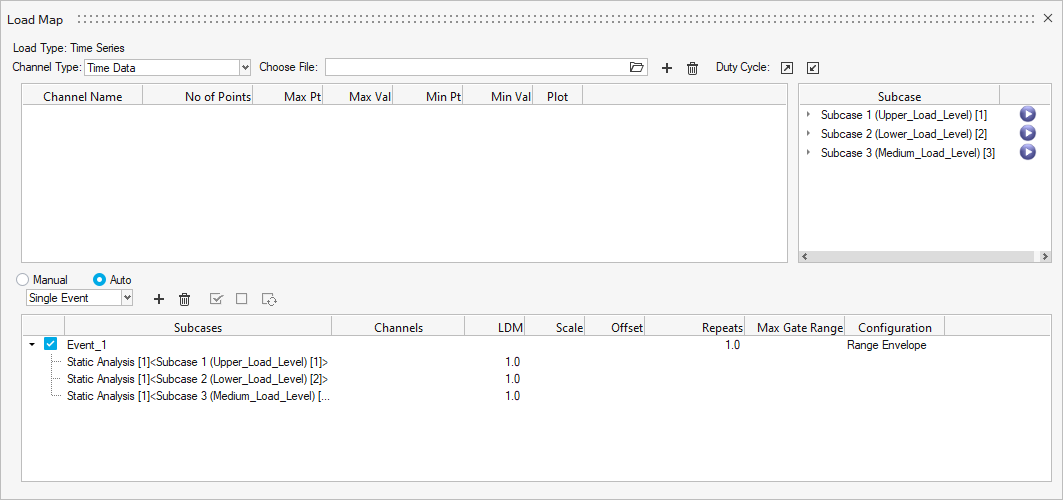## Create Duty Cycle Events

HyperLife facilitates in importing Duty Cycle files (*.dcy) which have complex duty cycle information for multiple durability events. HyperLife provides the following options in automatically creating the necessary events.
• Duty Cycle file (*.dcy)

## DCY File

Duty Cycle is supported for all fatigue calculations with a Time Series loading type.

1. Click the Load Map tool.
2. Select Duty Cycle from the Channel Type drop-down menu in the top-left of the dialog.
3. In the Choose file field, click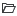and browse for a *.dcy file.
4. Click.
On importing the file:
• The load history files are imported into the channel window.
• Subcase and channel pairs are created in the event window.
• Event information specific to repeats, scale, LDM, and offset is updated.
Note: The load history files need to be present in the same directory as the *.dcy file.

The files formats supported for load history within the *.dcy file are: *.csv, *.rpc, *.rsp, and *.dac.

The key words from the *.dcy file define the required duty cycle information, such as:
• Channel number (Chan)
• Load history file name with extension (Testname)
• FE subcase ID in HyperLife (FELoadCase)
5. Optional: Update any parameters as needed or disable an event to exclude it from the solution.

Simulation list/increments within a FE subcase can be included in *.dcy event pairing as SubcaseID.Simulation.ID as shown in the example below.

## XML File

LoadMap file (*.xml) is supported for the following loading types: Time Series, Transient Response, Modal Superposition, Random (PSD Stresses), Random (Input PSD with FRF), and Sine Sweep.

1. Click the Load Map tool.
2. In the Load Map dialog, clickin the top-right.
3. From the file dialog, browse for an *.xml file and click Open.
On importing the file:
• The load history files are imported into the channel window.
• Subcase and channel pairs are created in the event window.
• Event information specific to repeats, scale, LDM, and offset are updated.
The key words from the *.xml files define the required duty cycle information, such as:
<LoadMap typeofloading="Time Series">
• Load History file name with extension
<Channel>
</Channel>
• Event information
<FatigeEvents>
<Event Configuration="superposition" Gate="0" id="1" name="Event_1">
</Event>
</FatigeEvents>
• Event sequence
<Fatseq>
<Fatevnt EventId="" EventName="Event_1" Repeats="1000"></Fatevnt>
<Fatevnt EventId="" EventName="Event_2" Repeats="5000"></Fatevnt>
</Fatseq>
• Load history file name with extension (Testname)
• FE subcase ID in HyperLife (FELoadCase)
4. Optional: Update any parameters as needed or disable an event to exclude it from the solution.

Simulation list/increments within a FE subcase can be included in *.xml event pairing sim = Simulation.ID as shown in the example below.

## Event Mapper CSV

Event Mapper file is an alternative to Duty Cycle file for when the Channel mapping doesn’t specifically follow a uniform order for each of the FE loadcases.

The Channel IDs (Time series data) and FE loadcase IDs are pre-sorted to form Events in a *.csv format, which is imported on Load Map utility.

### Terminology

• Channel Mapping: Concatenating Load history data together with corresponding FE loadcase to form a Durability Event.
• Durability Event creation is critical step and intense when large load history is considered.
• Need arises to consider fewer channels from a Load history file (for example, RSP) which is not in sequence.
• FE loadcase and Channel data don’t follow an order for the tool to do Auto pairing.
Note: Load history files should already be imported to the session prior to Event Mapper CSV.

## Create Transient Response Events

Transient subcases are supported for SN (uniaxial, multiaxial), EN (uniaxial, multiaxial), and FOS fatigue calculations with a Transient Response loading type.
1. Click the Load Map tool.
By default, Transient Response is the selected Channel Type and can not be changed.
Note: Plots or channels are not required.
2. Select a subcase from the Subcase panel then clickon the bottom half of the dialog to create an event.
Stresses from each time step of the subcase are superimposed and damage is calculated on the superimposed stress.
Note: You can only select one transient subcase per event.
3. Optional: Edit the number of repeats.
Tip: Apply a Repeats value to all the events by right-clicking on a value and selecting Apply value to all events.
4. Apply Max Gate Range ( (0.0 ≤ Real < 1.0), to apply gate on the Stress History.
5. Enable the checkboxes of the events to be considered in the evaluation.

## Create Modal Superposition Events

Modal subcases are supported for SN (uniaxial, multiaxial) and EN (uniaxial, multiaxial) fatigue calculations with a Modal Superposition loading type.

1. Click the Load Map tool.
2. Select the required modes from mrf/pch and the FE subcase.
3. On the bottom half of the dialog, select Auto and click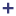.
A modal event is created.
4. Optional: Edit the number of repeats.
Tip: Apply a Repeats value to all the events by right-clicking on a value and selecting Apply value to all events.
5. Apply Max Gate Range ( (0.0 ≤ Real < 1.0), to apply gate on the Stress History.
6. Activate the checkboxes of the events to be considered in the evaluation.
Stress history from modal participation factors and modal stresses is calculated as:(4) ${\sigma }_{ij}\left(t\right)={\sum }_{k}{P}_{k}\left(t\right)*{\sigma }_{ij,k}$
Where,
${\sigma }_{ij}\left(t\right)$
Stress history for the given time interval of an element
${P}_{k}\left(t\right)$
Participation factor per mode at time t (via mrf/pch file)
${\sigma }_{ij,k}$
Modal stress of an element per mode (via an h3d or op2 file)
Note: op2 files are only supported for mrf channels. h3d is supported for both mrf and pch.
$k$
Mode

## Create Random (PSD Stresses) Events

Random Response subcases are supported for SN (uniaxial) and EN (uniaxial) fatigue calculations with a Random (PSD Stresses) loading type.

1. Click the Load Map tool.
By default, PSD Stresses is the selected Channel Type and can not be changed.
Note: Plots or channels are not required.
2. Select a subcase from the Subcase panel then clickon the bottom half of the dialog to create an event.
The Subcase panel lists random response FE subcases. FE static subcases are listed if mean stress correction is required.
Note: You can only select one random subcase per event.
3. Optional: Drag-and-drop one static subcase onto an event if mean stress correction is to be introduced.
4. Optional: Edit the Exposure Time value.
Tip: Apply an Exposure Time value to all the events by right-clicking on a value and selecting Apply value to all events.
5. Enable the checkboxes of the events to be considered in the evaluation.

## Create Random (Input PSD with FRF) Events

Frequency Response Analysis subcases are supported for SN (uniaxial) and EN (uniaxial) fatigue calculations with a Random (Input PSD with FRF) loading type.
Note: Default format on import of Input PSD is LINEAR - LINEAR (that is, linear interpolation for the x-axis and linear interpolation for the y-axis). X Scale and Y Scale is to be changed to the required format. For example: LOGARITHMIC - LOGARITHMIC.
1. Click the Load Map tool.
2. Select subcases.
3. On the bottom half of the dialog, clickto create an event.
The possible correlations (Excitation1 and Excitation2) of the selected FRF subcases are listed under the event.
4. Drag-and-drop the imported channels onto the Input PSD field of each correlation.
The Input PSDs are used to scale the complex stresses. Input PSD of frequency vs scale in CSV format are supported.
5. Scale the complex stresses using the Input PSD and the Real and Imaginary coefficients from each event.
6. Optional: Edit the Exposure Time value.
Tip: Apply an Exposure Time value to all the events by right-clicking on a value and selecting Apply value to all events.
7. Enable the checkboxes of the events to be considered in the evaluation.
Note:
• If Mean Stress correction is to be applied, a static subcase, if present in the result file, will be listed in the Subcase window and can be drag and dropped onto the event (no channel is required to be paired).
• Any events that you create are specific to the channel type. If you switch the channel type, the events window is cleared/updated.

## Create Sine Sweep Events

Frequency Response Function subcases are supported for SN (uniaxial) and EN (uniaxial) fatigue calculations with a Sine Sweep loading type.

1. Click the Load Map tool.
3. On the bottom half of the dialog, clickto create an event.